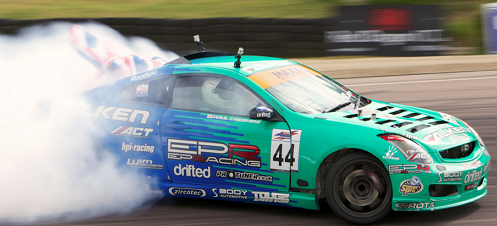# Help it turn!A car has to move on a level turn of radius 20m. The coefficient of static friction between the tires and the road is 2.00. Find the maximum speed the car can take without skidding.

Details and assumptions:

• $g = 10 \text{m/s}^2$

• Give your answer in $\text{m/s}$.

×